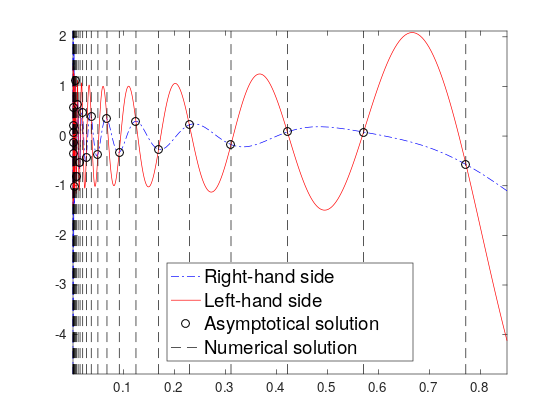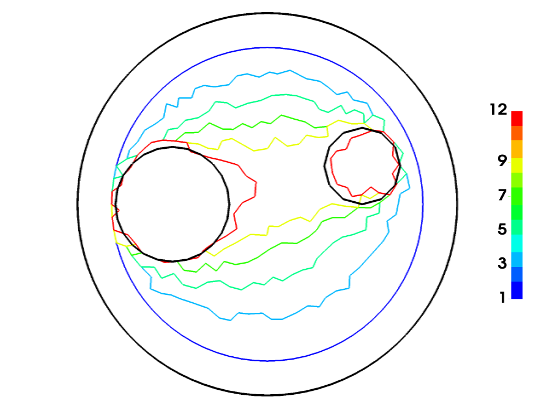Dr.  Dmitry PonomarevFinding eigenvalues as solution of asymptotical characteristic equationScaling for an NLS model of laser beam propagation in a photopolymerObstacle (2 black circles) identification: red curve is the last iterationCauchy problem for Laplace equation with "interior measurements"My analytical triumph for magnetization estimation in the set-up of EAPS lab, MITAn attack on the spectral problem for the truncated Poisson operator

I am an applied mathematician primarily interested in real-life problems whose treatment requires analysis of a model / justification of the approximation or those that can be effectively tackled with analytical tools such as asymptotic methods or closed-form solution reducibility.

I enjoy learning new things while working on diverse subjects analyzing a problem, devising a constructive solution approach and verifying / implementing it numerically. The topics I have previously worked on include:

• wave propagation in porous media (fluid / solid inclusion scattering for Biot elastodynamics equations);
• nonlinear PDEs (analysis of one NLS model for laser beams in photopolymers; Darboux transformation);
• approximation theory (approximation of square-integrable functions by traces of analytic functions with certain properties such as pointwise constraints);
• integral equations and optimal bases construction (spectral theory for compact one-dimensional self-adjoint integral operators with convolution kernels);
• inverse magnetization problem (analytical estimation of net magnetization moment components from partial measurements of magnetic field);
• inverse obstacle problem (determining domain geometry for transient wave equation from partial Dirichlet-Neumann data).

At the present time, I am a postdoctoral researcher at Insitute of Analysis and Scientific Computing, TU Wien, Austria.
Together with Anton Arnold, we are developing hybrid asymptotical-numerical methods for efficient treatment of high-frequency problems for wave equations.

You can contact me by email: dmvpon@gmail.com / dmitry.ponomarev@asc.tuwien.ac.at, or find me at:
Office DA 06 L14, Institute of Analysis & Scientific Computing, Vienna University of Technology, Wiedner Hauptstrasse 8, Vienna, Austria.